# Potential and Kinetic Energies in Simple Harmonic Motion

We have read that a particle executing simple harmonic is acted upon by a restoring force, which tends to bring back the particle to its mean position. Hence the particle possesses the =’Potential energy. Since the particle remains in motion, hence it also possesses the kinetic energy.

The two forms of energy transform in each other during the motion, but their sum always remains constant.

Potential Energy

Let at any instant t, the displacement of particle of mass m from its mean position be x at that constant, the acceleration of particle be f Then in simple harmonic motion, acceleration f α – x

f = – ω2x, where ω2 is a constant

:. The restoring force acting on the particle is

F = mass x acceleration = mf = – m ω2x

Here the negative sign only gives the direction of force which is opposite to the direction displacement. Obviously, the magnitude of force is proportional to the displacement.

This Work done against the restoring force in displacing the particle from x to (x + dx) is   dW = m ω2x dx.

This work done is -stored in the particle in. the form of potential energy. Hence total work done in displacing the particle from mean position x = 0 to displaced position x = potential energy of the particle at displacement x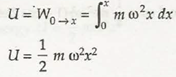Kinetic Energy

From equation (2) of article 1.3, at an instant t, when the displacement of particle is x, the velocity of particle is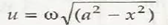Kinetic energy of particle K = 1/2 mu2

K =1/2  m w2(a2 – x2)

Total Energy

Total energy of particle = Potential energy + Kinetic energy

=1/2 mwx2 +1/2 mw2 (a2-x2)

= 1/2  m w2a2

But w = 2πn (where n is frequency)

[ E = 2π2m  n2a2]

From the above equation (3), we conclude that

(i) The total energy of the particle executing simple harmonic motion does not depend on its displacement x and time i.e. during the motion, the total energy of the particle foes not change with time (or position).

(ii) In the extreme position (x =±a), kinetic energy is zero, hence the total energy is in form of potential energy.

(iii) In mean position (x = 0), potential energy is zero, hence total energy is in form of kinetic energy.

(iv) As the displacement of particle from its mean position increases, its kinetic energy decreases and potential energy increases.

Similarly, as the particle approaches from the extreme position to the mean position, its · potential energy decreases and kinetic energy increases, but in ideal condition when there is no loss of energy due to friction etc. in any position, the total sum of kinetic energy and potential energy remains constant, i.e., the mechanical energy remains conserved.*

(v) Total energy of the particle executing simple harmonic motion is directly proportional to the square of amplitude of motion i.e., E r a2.

(vi) For the particle executing simple harmonic motion, is directly proportional to the square of frequency of motion i.e., E r n2

(vii) For a particle executing simple harmonic motion, the average kinetic energy is equal to average potential energy and it is equal to half of the total energy i.e.,

Kau = Uar, = 1/2 E

The average kinetic energy of a particle in one period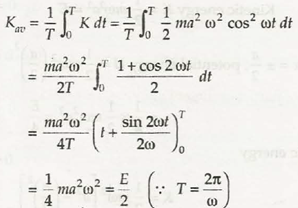The average potential energy of a particle in one time period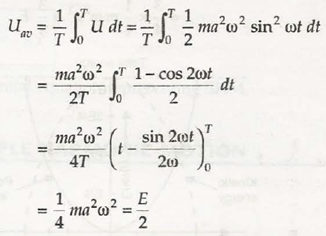It is clear from equations (4) and (5), that Kav = Uav = E/2

Or [k av +Uav =E]

Graphical Representation of Potential Energy and Kinetic Energy

Let the displacement of particle of mass m executing simple harmonic motion at any instant t be x, then in that position of potential energy and kinetic energy of particle are given as

Potential energy U= ½ mw2x2

and

and                  kinetic energy K = 1/2 mw2 (a2 – x2)

where a is the amplitude of motion and w the angular velocity

Total energy               E = U + K = 1/2 mw2a2

(i) When x = 0(i,e., at mean position)  potential energy U = 0

Kinetic energy K =1/2  mw2a2 = E

(ii) When X= ± a/2 , potential energy u       =1/2  mw2 (a/2)2

= 1/4 x 1/2  mw2 a2 = E/4

and kinetic energy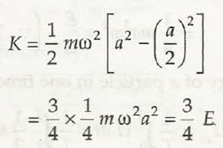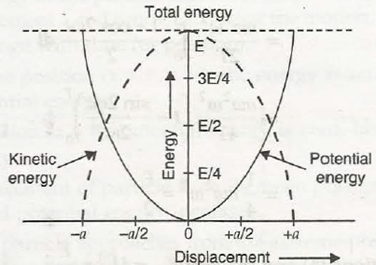FIGURE 1.3 Graphical representation of potential energy and kinetic energy in simple harmonic motion

(iii) When x = ± a, potential energy U = 1/2 m w2-a2 = E

kinetic energy K = 0

Now taking the displacement x on X-axis and the energy on Y-axis, the graph plotted is shown in Figure 1.3.

The dotted line drawn parallel to X-axis represents the total energy of the particle, which remains constant during the entire motion.